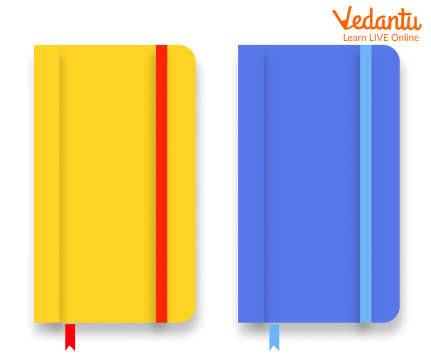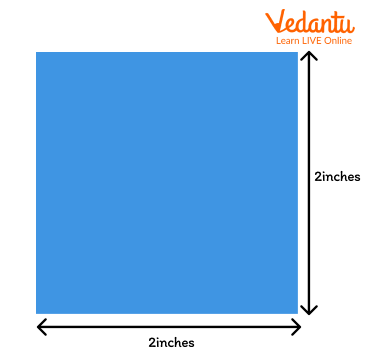Courses
Courses for Kids
Free study material
Free LIVE classes
More

# What are Attributes in Math?## Attributes - An Assistant for Mathematics Study and Calculation

Maths involves accurate noticing of the traits or properties of a shape or an object at hand. These traits and properties of a particular object or shape are called its attributes.

Attributes, thus, become very important for mathematical study as well as for any mathematical calculation.

What are attributes in Maths? We will learn this more clearly in this content. Here we will be discussing the attributes that should be considered in Maths.

### What do You Mean by Attributes?

‘Attributes’ in the study of Mathematics means the traits and properties of a particular object. Let us consider an example to explain more precisely.

Examples

Suppose we have a simple Mathematics calculation, where we need to find out the sum of numbers. In this scenario, first, we will look at the type of addition required, if it is a three-block addition or a two-block, or simple one-block addition. Then we will check for any decimal places, after these analyses we proceed on with the sum. This checking of the number of blocks in addition or the form of a number in the question is the attributes of the particular sum.

Let us simplify even further, from the following diagram, observe the properties of both the books -Two books - Yellow and Blue

The properties of the books can be:

1. The shape of the book is rectangular.

2. The colours of the book are yellow and blue.

3. We will check the page count in the book.

4. The length and width of both the books will also be checked.

5. We will take note of the weight of the book as well.

Thus these are the attributes of the books which can be used for calculation of the area covered by the book or other such mathematical calculations.

### Attributes of Geometrical Shape

Since Geometry is another branch of Mathematics, the attributes of the geometric shapes work well. Moreover, the attributes of a geometric shape define the characteristics of that particular shape in terms of our imaginary vision which are required to be dealt with mathematically.

In the following diagram, we have the shape of a square:A square with sides 2 inches

Now, there are two types of attributes - Visual Attributes and Mathematical Attributes. Visual attributes are those attributes that are perceived via vision simply. While mathematical attributes are those attributes that are required in mathematical calculations.

The visual attributes of the square in the above diagram are:

1. The shape has 4 sides and 4 vertices.

2. All the sides of the square are equal.

3. The opposite sides of the square are also parallel in nature.

Now, let us look at the Mathematical attributes of the square in the above diagram:

1. The sides of the square measure 2 inches each.

2. The angles which are present on two adjacent sides sum up to 90 degrees.

### Attributes Help us Identify the Type of Triangles

Attributes help us in identifying many shapes and objects. Similarly, these attributes also help us in identifying the type of triangles too. Did you know? There are three types of triangles:

1. Right Angle Triangle

2. Equilateral Triangle

3. Scalene Triangle

In a right-angled triangle, one of the angles is 90 degrees. In an equilateral triangle, all the sides are of equal measurement. While, in a scalene triangle, one of the angles is more than 90 degrees.

So, these attributes and properties of triangles differentiate each other and make them unique.

### Did You Know?

1. The famous star constellation after the Zodiac signs is founded by the means of attributes.

2. Many computer programming languages and coding involve the usage of functions and attributes.

In this study, we have gained knowledge about the importance of attributes in Maths. In elementary schools, the kids learn and initiate visual observation priorly to any complicated calculations. Visual attributes are indeed the first step in learning the attributes. They visualize the mathematical operators, symbols, and numbers. Thus, we see attributes are involved in Maths right from elementary school. So, the students must be made aware of this gradually.

Last updated date: 20th Sep 2023
Total views: 137.7k
Views today: 1.37k

## FAQs on What are Attributes in Math?

1. What do you mean by pattern attributes in Maths?

To work with the shapes and objects, the children are required to observe the shape, colour, sound, texture, objects, and action. These are called pattern attributes.

2. What are the attributes of a rectangle?

The attributes of a rectangle are as follows:

• This is a 2-D shape in geometrical mathematics.
• They have 4 sides and 4 corners.
• The sides meet and make 90 degrees.
• The opposite sides of the rectangle are equal and they are parallel to each other.